# python 實現 2048 游戲 （二）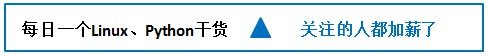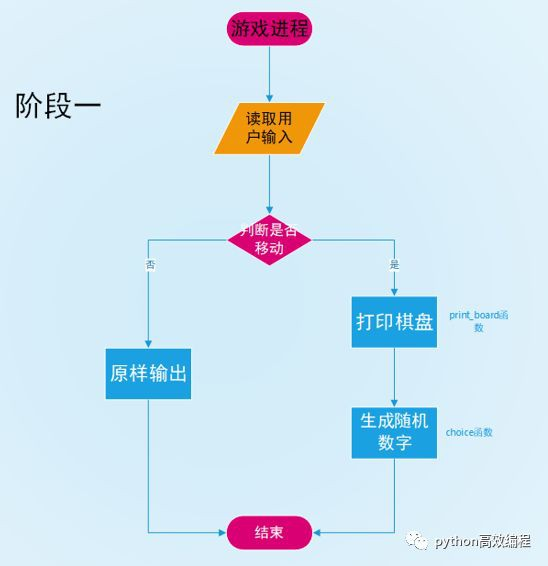• 用戶輸入小寫的 w s a d 對應上下左右

• 該移動方向上允許移動

``````def game(board, stdscr, rscore):
global score
global change

# curses.noecho()
# 屏幕不顯示用戶輸入的字符
curses.noecho()
while 1:

# stdscr.getch()
# 讀取用戶輸入的字符
order = stdscr.getch()

# move()對用戶移動的響應
current_board, change = move(order, board)
# change 為 1 隨機產生 2 或 4
if change:
current_board = choice(board)

# 打印棋盤
print_board(stdscr, current_board, rscore)

# 當棋盤被填滿，判斷是否游戲結束
if (current_board != 0).all():
fail(current_board)

# win 為 1 打印獲勝提示
if win:

basic 函式用來執行移動與碰撞的操作。move_{up,down,right,left} 函式用來實現各個方向上的 basic 函式操作。move 函式用來響應用戶指令，實現各個方向上的移動。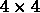矩陣

• 4 與 0 不交換             [2 2 0 4]

• 0 與 2 交換                 [2 0 2 4]

• 0 與 2 交換                 [0 2 2 4]

• flag = 1 且 score + = 0

• 4 與 2 不交換             [0 2 2 4]

•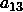雙倍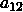置 0      [0 0 4 4]

• 0 不變                        [0 0 4 4]

• flag = 1 且 score += 100

•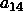雙倍置 0     [0 0 0 8]

• 不變                          [0 0 0 8]

• 不變                          [0 0 0 8]

• flag = 1 且 score += 100

• 不變

• 不變

• 不變

• flag = 0 且 score += 0

``````# A 為 4*4 的矩陣
# 轉置操作
A.T
# 逆置操作
A[::-1,::-1]``````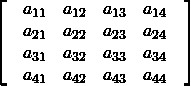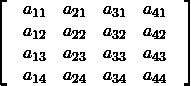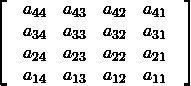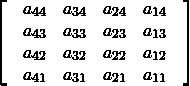``````# 基礎移動
def basic(board):
global score
global win
# 以右移為基礎移動

for i in range(4):
flag = 1
while flag:
flag = 0
j = 2
while j >= 0:
if board[i, j] != 0:
if board[i, j + 1] == board[i, j]:
board[i, j + 1] = 2 * board[i, j]
if board[i, j + 1] == 2048:
win = 1
board[i, j] = 0
score += 100
flag = 1

elif board[i, j + 1] == 0:
temp = board[i, j]
board[i, j] = board[i, j + 1]
board[i, j + 1] = temp
flag = 1

j -= 1
return board

# 右移
def move_right(board):
return basic(board)

# 上移
def move_up(board):
# 逆置 + 轉置
board = board[::-1, ::-1].T
board = basic(board)
board = board[::-1, ::-1].T
return board

# 左移
def move_left(board):
# 逆置
board = board[::-1, ::-1]
board = basic(board)
board = board[::-1, ::-1]
return board

# 下移
def move_down(board):
# 轉置
board = board.T
board = basic(board)
board = board.T
return board

# 移動
def move(order, board):
# ord 求碼值
global score
global win
change = 1
tempboard = copy.deepcopy(board)

# 退出游戲
if order == ord('q'):
save_score(score)
exit()
# 重置游戲
elif order == ord('r'):
win = 0
save_score(score)
score = 0
stdscr.clear()
wrapper(main)
# 勝利後，只有退出和重置游戲
elif win:
change = 0
newboard = tempboard
return newboard, change
# 上下左右移動
elif order == ord('w'):
newboard = move_up(board)
elif order == ord('s'):
newboard = move_down(board)
elif order == ord('a'):
newboard = move_left(board)
elif order == ord('d'):
newboard = move_right(board)

# 按其他鍵程式不響應
else:
newboard = board

if (newboard == tempboard).all():
change = 0

return newboard, change``````

``````# 隨機產生 2 或 4
def choice(board):
udict = {}
# 統計0的個數
count = 0
for i in range(4):
for j in range(4):
# board[i,j] 為 0
# eg. {0:(1,3),1:(2,1),3:(3,2)}
# 根據 key 可以獲得元素 0 在棋盤上的位置
if not board[i, j]:
udict[count] = (i, j)
count += 1
# np.random.randint(0, count)
# 產生 [0,count) 範圍內的隨機數
random_number = np.random.randint(0, count)
# np.random.choice([2,2,2,4])
# 隨機選取串列 [2,2,2,4] 中的元素
two_or_four = np.random.choice([2, 2, 2, 4])
# 更改棋盤上 0 元素為隨機數
board[udict[random_number]] = two_or_four
return board``````

``````# 加載最高分
return rank_score

# 儲存最高分
def save_score(score):
if score > rscore:
np.save(FILENAME, score)

# 比較當前分數與當前最高分
def compare_score(score, rscore):
if score > rscore:
rscore = score
return rscore``````

``````# 打印棋盤
def print_board(stdscr, board, rscore):
global score
rscore = compare_score(score, rscore)

# stdscr.clear()
# 清除屏幕
# 打印字串
stdscr.clear()
for i in range(4):
for j in range(4):
if board[i, j]:
else:

``````import numpy as np
import curses
import copy
import os
from curses import wrapper

stdscr = curses.initscr()
# 分數
score = 0
# 判斷是否獲勝
win = 0
#
FILENAME = 'out.npy'

# 初始化
def init():
# 初始化棋盤
# 初始棋盤 2 或 4 的隨機數字
if FILENAME not in os.listdir():
np.save(FILENAME, 0)
init_board = choice(np.zeros((4, 4), dtype=np.int))
return init_board

# 主程式
def main(stdscr):
# 初始化程式
init_board = init()